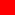## KALYAN CITY LIFE

Sharing Wisdom and Vivid Memories of Life

# Simple JavaScript Calculator Code - JavaScript Arithmetic

##Write HTML Code For Simple JavaScript Calculator

Yesterday I revised the concept of HTML Forms and JavaScript. While revising I encountered a University question stating, "Q. Write A HTML Code To Make Simple Calculator Using Javascript Functions and HTML Forms. Calculator Must Take Any Two Numbers from User and Perform 5 Basic Arithmetic Operations i.e Addition, Subtraction, Multiplication, Division and Modulo".

I gave it a try to write this small calculator application (using Javascript and HTML Forms) and managed to produce following working script. This Javascript application is very simple, necessarily commented and is easy to understand. I hope it may prove useful to those interested in learning javascript functions, hence feel like sharing. To understand more, How Javascript Works? I recommend you refer this JavaScript Tutorial.

##JavaScript Code - Simple JavaScript Calculator

```<html>

<title>Simple Javascript Calculator - Basic Arithmetic Operations</title>

<!-- Aim: Write HTML Code Using JavaScript Functions To Perform Basic Arithmetic Operations. -->
<!-- 1. Take Two numbers from user say 'Number 1' and 'Number 2'. -->
<!-- 2. Perform Addition, Subtraction, Multiplication, Division and Modulus. -->
<!-- 3. Result must be displayed on same HTML Page when respective button is clicked. -->
<!-- 4. Use <input> tag (HTML Forms Concept) with onclick.-->
<!-- 5. Call individual Javascript Function, put them inside <head> tag only.-->
<!-- 6. Javascript Tutorial/Code For Computer Science Students. -->
<!-- 7. Tested and Written By (c) Gaurav Akrani. -->

<script language="javascript" type="text/javascript">
function multiply(){
a=Number(document.calculator.number1.value);
b=Number(document.calculator.number2.value);
c=a*b;
document.calculator.total.value=c;
}
</script>

<script language="javascript" type="text/javascript">
a=Number(document.calculator.number1.value);
b=Number(document.calculator.number2.value);
c=a+b;
document.calculator.total.value=c;
}
</script>

<script language="javascript" type="text/javascript">
function subtraction(){
a=Number(document.calculator.number1.value);
b=Number(document.calculator.number2.value);
c=a-b;
document.calculator.total.value=c;
}
</script>

<script language="javascript" type="text/javascript">
function division(){
a=Number(document.calculator.number1.value);
b=Number(document.calculator.number2.value);
c=a/b;
document.calculator.total.value=c;
}
</script>

<script language="javascript" type="text/javascript">
function modulus(){
a=Number(document.calculator.number1.value);
b=Number(document.calculator.number2.value);
c=a%b;
document.calculator.total.value=c;
}
</script>

<body>

<!-- Opening a HTML Form. -->
<form name="calculator">

<!-- Here user will enter 1st number. -->
Number 1: <input type="text" name="number1"> <br>

<!-- Here user will enter 2nd number. -->
Number 2: <input type="text" name="number2"> <br>

<!-- Here result will be displayed. -->
Get Result: <input type="text" name="total"> <br>

<!-- Here respective button when clicked, calls only respective artimetic function. -->
<input type="button" value="SUB" onclick="javascript:subtraction();">
<input type="button" value="MUL" onclick="javascript:multiply();">
<input type="button" value="DIV" onclick="javascript:division();">
<input type="button" value="MOD" onclick="javascript:modulus();">

</form>

</body>
</html>
```

##JavaScript Output - Perform Arithmetic Operations

Copy and paste above HTML Code in a notepad and save it as JavaScript-Calculator.html. Now open this saved HTML file in any web browser (e.g: Internet Explorer with ActiveX Enabled) to get result something like this...

"Thanks! For reading my JavaScript Tutorial. Enjoy Scripting"...Gaurav Akrani.

## Sitemap of Kalyan City Life Blog

1. Anonymous said...

its a perfect code for simple calculator in java script,,,

2. Zed said...

Just fantastic, 5 STARS
simple and well explained thank you very much
for someone like me who's new to JavaScript, this was very helpful

3. Anonymous said...

A good template for programmable calculating.

4. RafaelJ3D said...

Hi,
I'm physics teacher and I want to know if
Could I use this code for create modify versions with my students?

Thanks,
Rafael

5. Gaurav Akrani said...